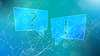# TechTopics No. 39

Heat generation estimation for type NXAIR P switchgear (up to 15 kV)

Siemens is often asked to provide estimated heat generation data for our equipment. This issue of TechTopics provides information that allows calculation of approximate heat generated by the switchgear under assumed loading conditions.

The heat generation data given in the table below is on the basis of full-rated continuous current. Actual heat generation calculations must take into account the true loading of the equipment.

The amount of heat generated is related to the square of the current, so a circuit breaker operating at one-half rated current will have heat generation only one-quarter of that at full-rated continuous current. Because the effect of the square relationship is very significant, it is overly conservative to estimate heat generation based on the assumption that all sections and all circuit breakers each carry their rated continuous current at all times. Air conditioning systems sized based on such estimates will be larger than the real operating conditions require.

 Rated current Actual current Circuit breaker cell with circuit breaker Forced air cooling fan (4,000 A circuit breaker cells only) 1,200 A 2,000 A 3,000 A 4,000 A 1,200 A 2,000 A 3,000 A 4,000 A 605 W 1,350 W 2,250 W 3,500 W ---- ---- ---- 75 W 195 W 250 W 350 W 550 W 200 W 100 W plus 100 W for each rollout tray 50 W 4% of CPT kVA rating 50 W

Notes on assumptions:

1. Space heaters, when provided, are not normally controlled by a thermostat; hence, their load is represented as a continuous load. The purpose of space heaters is to prevent condensation, and this is not limited by the absolute temperature. Even when a thermostat is used to control the heaters, it is set to shut the heaters off at a temperature of approximately 110 ºF. Therefore, in an air-conditioned room, the heaters would be energized continuously.
2. Heat generated by current transformers is ignored as it is usually insignificant, and varies according to the CT ratio, as well as the loading. The data above includes the maximum number of CT cores per circuit breaker compartment.
3. The CPT heat generation estimate is very conservative and assumes that the CPT is operated at full-rated capacity. If normal loading is at less than full rating, heat generation may be adjusted by the square of the percent loading.
4. Relaying and instrumentation heat generation estimates are very approximate. Extensive relaying and instrumentation may warrant additional conservatism in the estimation of associated heat generation.
5. Conversion factor: watts x 3.415179 = BTU/hour.

To estimate the heat generated under actual loading conditions, determine the component heat generation for each of the components indicated in the table. Estimated heat generation for circuit breakers should be adjusted for actual loading based on the ratio of the squares of the actual current and the rated current. To be precise, this adjustment should also be made for the actual current loading for each individual vertical section, but this is frequently ignored in the interests of simplification. Instead, the main circuit breaker loading is usually assumed to be equal to the main bus loading in all vertical sections.

Example: Assume a lineup with six vertical sections, one 3,000 A main circuit breaker (loaded to 2,150 A), four 1,200 A feeder circuit breakers (loading 850 A, 250 A, 600 A and 450 A), with 3,000 A main bus, and including space heaters. The lineup includes one VT rollout, one 10 kVA CPT rollout, and microprocessor relaying and instrumentation. The calculations would be as follows:

Table 2: Example
Item
Category
Heat generation
Heat generation
A
3,000 A circuit breaker at 2,150 A = 2,250 x (2,150/3,000)2 =
1,155 W
----
A
1,200 A circuit breaker at 850 A = 605 x (850/1,200)2 =
304 W
----
A
1,200 A circuit breaker at 250 A = 605 x (250/1,200)2 =
26 W
----
A
1,200 A circuit breaker at 600 A = 605 x (600/1,200)2 =
151 W
----
A
1,200 A circuit breaker at 450 A = 605 x (450/1,200)2 =
85 W
----
A
Total heat generation, circuit breaker cells
----
1,721 W
B
Forced air cooling fan (4,000 A circuit breaker cell only)
----
----
B
Vertical sections with 3,000 A bus at 2,150 A = 6 x 350 x (2,150/3,000)=
----
1,079 W
C
Space heaters for 4 circuit breaker cells = 5 x 200 =
----
1,000 W
C
Space heaters for 1 auxiliary cell with two rollouts = 100 + (2 x 100) =
----
300 W
D
VT rollout = 1 x 50 =
----
50 W
E
CPT = 1 x 4% x 10 kVA =
----
400 W
F
Relaying and instrumentation = 5 x 50 =
----
250 W
Total
Total estimated heat generation under assumed loading conditions
----
4,800 W

If true loading were not considered (e.g., all calculations performed on the basis of full rated current), the calculations would yield a heat generation of 8,770 watts, nearly double the “real” heat generation.